Courses

# Generalized Correlations for Computing dH and dS for a Real Gas Civil Engineering (CE) Notes | EduRev

## Civil Engineering (CE) : Generalized Correlations for Computing dH and dS for a Real Gas Civil Engineering (CE) Notes | EduRev

The document Generalized Correlations for Computing dH and dS for a Real Gas Civil Engineering (CE) Notes | EduRev is a part of the Civil Engineering (CE) Course Thermodynamics.
All you need of Civil Engineering (CE) at this link: Civil Engineering (CE)

The approach based on the use of the compressibility factors can be applied to the present instance to evolve generalized correlations for computing enthalpy and entropy changes for gases. We start with the Pitzer-type expression for the compressibility factor:

Z =Z0 +ω Z                     ..(2.25)

Differentiating with respect to the reduced temperature we get: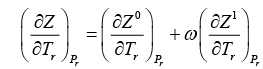..(5.55)

Thus using eqn. 5.54 we may recast eqns. 5.37 and 5.38 as follows: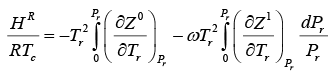..(5.56)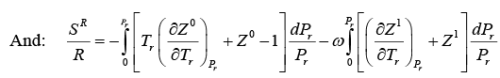..(5.57)

Both the above equations may be rewritten individually as follows: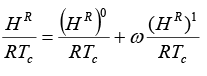..(5.58)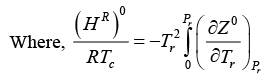..(5.59a)

And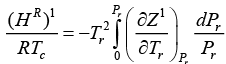..(5.59b)

Similarly: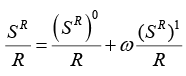..(5.60)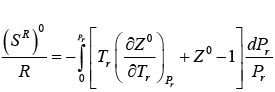..(5.61a)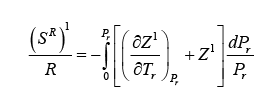..(5.61b)

The term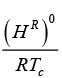in eqn. 5.59a constitutes the first order enthalpy departure, and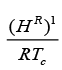(in eqn. 5.59b) the second order (with respect to simple fluids) enthalpy departure at specified Tr and Pr. The same is true for the corresponding entropic terms provided by eqns. 5.61a and 5.61b.

The evaluation of the integrals in eqns. 5.59 to 5.62 may be carried out assuming an EOS. The most widely used approach is that of Lee and Kesler who employed a modified form of the BWR EOS (eqn. 2.17) to extend their generalized correlation to residual property estimation. Figs. 5.1 and 5.2 respectively provide values of (HR)0/RTC an (HR)1/RTC respectively. Similar plots for the entropy terms (SR)0 / R, an ( SR)1 /R are also available; however, here the plot of the entire entropy term (− SR) as function of Tr and Pr is shown in fig. 5.3. Table of values of all the above (eqns. 5.59 to 5.62) are also available as functions of T r and P r Fig. at discrete intervals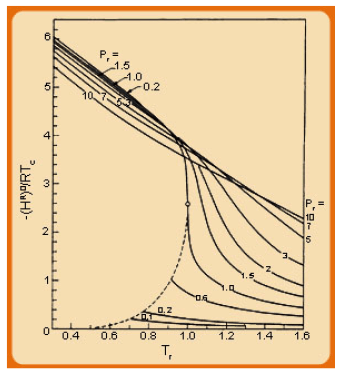Fig. 5.1 Correlation of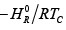drawn from tables of Lee-Kesler (Source: AIChE  J., pp. 510-527, 1975)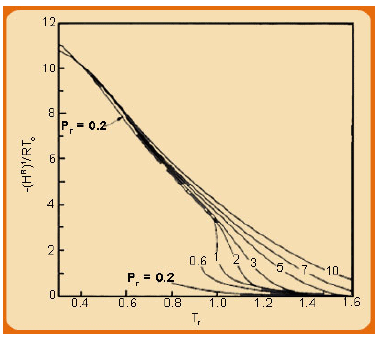Fig. 5.2 Correlation of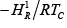drawn from tables of Lee-Kesler (Source: AIChE  J., pp. 510-527, 1975)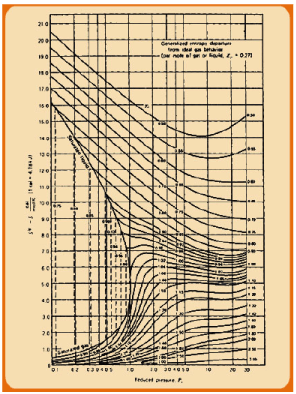Figure 5.3 Generalized entropy departure functions using corresponding states.

Offer running on EduRev: Apply code STAYHOME200 to get INR 200 off on our premium plan EduRev Infinity!

## Thermodynamics

56 videos|92 docs|33 tests

,

,

,

,

,

,

,

,

,

,

,

,

,

,

,

,

,

,

,

,

,

;